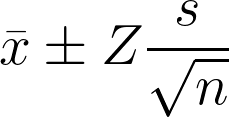# 90% Confidence Interval Calculator

Created by Aleksandra Zając, MD
Reviewed by Krishna Nelaturu
Last updated: Oct 11, 2022

If you're facing a statistics problem finding a 90% confidence interval for your sample, this site is the right place! Our 90% confidence interval calculator will help you determine that range in the blink of an eye. Read on to find out:

• How to find a 90% confidence interval;
• What is z-score for 90% confidence interval (Z(0.90)); and
• How to use our tool!

## What does a 90 percent confidence interval mean?

Let's understand what does 90% confidence interval mean before we dig deeper into calculating one.

Imagine Joe, who has an apple orchard and sells boxes of apples. He wants to be loyal to his clients and check how much the full boxes weigh. The label says 18 kg (39.68 lb), but what's the reality? He measured the consecutive 170 boxes that came out of his orchard. He found out the mean weight of a single package was 18.02 kg, and he calculated the 90% confidence interval between 17.63 kg and 18.41 kg. How can we interpret those results? When Joe sells boxes of apples, he can be 90% sure that a single box weighs between 17.63 and 18.41 kg. He's a loyal seller, indeed.

## How to use the 90% confidence interval calculator?

Let's use our tool together, step by step.

1. Enter the mean (average) of your sample (x̅).
2. Fill in the standard deviation (s).
3. Type in your sample size - number of measurements taken (n).
4. You don't have to manually enter the confidence level, as there is a 90% set already. Keep in mind that you can change it anytime.
5. You don't need to type in the z-score value. This row automatically reacts to the confidence interval row and will change accordingly.
6. Your results are ready. You can now take a look at:
• The chart describing your data;
• The upper bound of the desired confidence interval;
• The lower bound of the confidence interval; and
• The margin of error.

## How do I find a 90% confidence interval?

So you'd like to step off the easy path and count the confidence interval on your own? Follow those steps:

1. Make sure you know three important values:

• $n$ - number of measurements (the size of the sample);
• $SE$ - the standard error;
• $σ$ - the standard deviation.
2. Calculate the $SE$ - standard error with the formula:

$\qquad SE=\frac{σ}{\sqrt{n}}$
1. Using standard error, we can estimate the $ME$ - margin of error.
$\qquad ME = SE × Z(0.90)$

Where $Z(0.90)$ is the z-score for 90% confidence interval. It is a fixed value that we take from the statistical table. Z-score for 90% confidence interval is equal to 1.645.

1. The only thing left is performing proper addition and subtraction to count your confidence interval's upper and lower bound of your confidence interval.
$\qquad upper\ bound = μ + ME$
$\qquad lower\ bound = μ - ME$

## More confidence interval tools

Check the rest of our confidence interval tools:

## FAQ

### How do I calculate a 90% confidence interval?

To count the 90% confidence interval:

1. First, calculate the standard error (SE) and the margin of error (ME).
SE = σ/√n
ME = SE × Z(0.90)
where σ is the standard deviation, n - sample size, Z(0.90) - z-score for 90% confidence interval.
2. Then determine the confidence interval range, using ME and μ - the calculated average (mean).
upper bound = μ + ME
lower bound = μ - ME

### What is the z-score for a 90% confidence interval?

Z-score for 90% confidence interval, or Z(0.90), equals 1.645.

Aleksandra Zając, MDSample mean (x̅)
Standard deviation (s)
Sample size (n)
Confidence level
%
or Z-score (Z)
σ90% of samples contain the population mean (μ) within the confidence interval x̅ ± E.
Confidence interval
Lower bound
Upper bound
Margin of error (E)
People also viewed…

### Black Friday

How to get best deals on Black Friday? The struggle is real, let us help you with this Black Friday calculator!

### Frequency distribution

The frequency distribution calculator generates the cumulative frequency distribution table and a bar graph representing the frequency distribution for the given set of numbers.

### Permutation

Use the permutation calculator to determine the number of permutations in a set.

### Pizza size

This calculator will help you make the most delicious choice when ordering pizza.